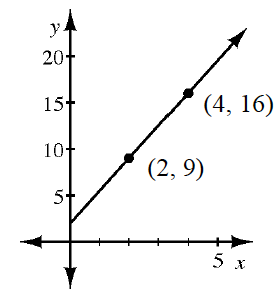### Home > CCA2 > Chapter A > Lesson A.2.3 > ProblemA-79

A-79.

Draw a slope triangle and use it to find the equation of the line shown in the graph at right.

$y=\frac{7}{2}x+2$